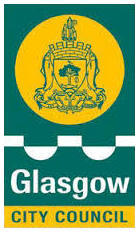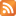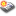##Welcome to Lourdes Primary School

Helping at Home with Number Stories

Helping at Home with Number Stories
Why do children need to know their number stories?
Children begin to see how smaller numbers are parts of larger numbers that when combined can help them to add and subtract more efficiently.
For example the story of 3        3+0=3    2+1=3     1+2=3     0+3=3
Story of 5       5                     5                              5                              5                              5
4   +    1            3    +   2                2   +    3                     4 + 1                       0 + 5
Children who have a good understanding of this can then very quickly understand that
2+1=3     1+2=3    3-1=2     3-2=1 etc
Activities at home
Finger Patterns-
Start with using just one hand. Ask your child to hold up 1 finger then 2,3,4,5. Try this with the numbers in order then try with the numbers all mixed up eg 3,5,2,4,1.
Now ask them to show you 1 using two hands. They will show one finger on one hand and clench their fist on the other hand to show 0.
Now continue with 2 and ask them to show you different ways-2+0, 1+1, 0+2.
Now move onto show me 3,4 and 5. When your child is confident, mix the numbers up eg show me 3,5,2,4,1.
Once your child is confident with numbers from 1-5 then move onto 6-10, using two hands and showing you lots of different ways to make each number.

Math Game: How Many Am I Hiding?

Materials: Any small objects that can be hidden behind your back or under a table, such as pennies, buttons counters or lego.
After playing with 2 you can decide if you need to have a smaller or larger amount of items.
1. Show the total amount of items to your child.
2. Place some of the objects behind your back. Remind them how many in all you started with.
3. Show your child the items not hidden.
4. Have them figure out how many are hiding behind your back.
5. Ask them to explain their thinking.
6. Show them the items that you have hidden and encourage them to check their answer.
7. Repeat the steps. When you feel your child masters the number, you can start to use bigger numbers.

Number Stories Quiz

Take one of the number stories that your child is working on eg the story of 10.

10+0=10
9+1=10
8+2=10
7+3=10
6+4=10
5+5=10
4+6=10
3+7=10
2+8=10
1+9=10
10+0=10
Show your child the number story and discuss any patterns that you see.
Now hide the number story and ask your child fill in the missing number 10+?=10, 5+?=10 etc.
Also try ?+4=10 , ?+7=10
If your child is having difficulties give them cubes, counters or pennies to help them work it out.
If they are getting the right answers confidently move onto the next number story.
OptionsRSS feed for this pageView Archive